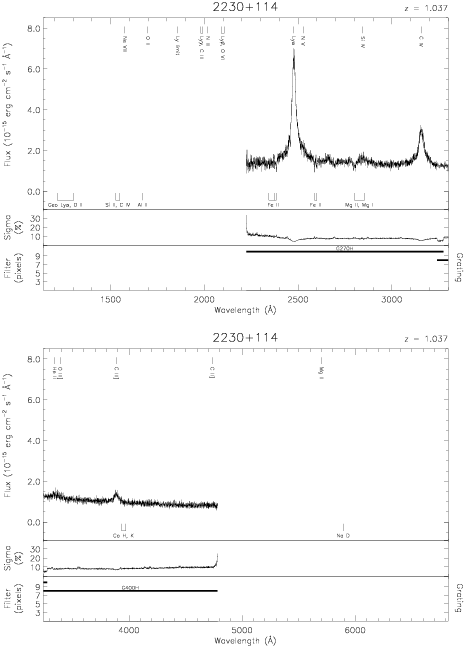Download dataset
TELESCOP= 'HST     '
INSTRUME= 'FOS     '
EQUINOX =               2000.0
DATE-OBS= '1991-09-12T03:31:19'
RA_PNT  =       338.1518750000
DEC_PNT =        11.7307500000
PA_PNT  =       241.8683000000
OBJECT  = '2230+114'
ROOTNM01= 'Y0PE0N02T'
GRNDMD01= 'SPECTROSCOPY'
DETECT01= 'AMBER   '
APERID01= 'A-1     '
FGWAID01= 'H27     '
ROOTNM02= 'Y0PE0N03T'
GRNDMD02= 'SPECTROSCOPY'
DETECT02= 'AMBER   '
APERID02= 'A-1     '
FGWAID02= 'H40     '